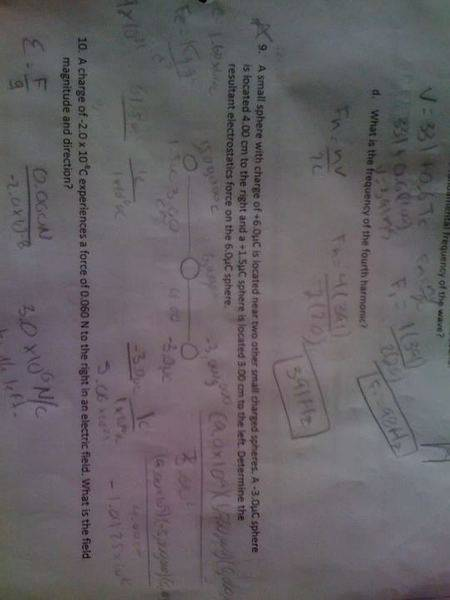# Coulombs Law solving for electrostatics force

## Homework Statement

A small spehere with charge of +6.0uc is located near two other small charged spheres. A -3.0uc sphere is located 4.00 cm to the right and a +1.5uc sphere is located 3.00 cm to the left. Determine the resultant electrostatics force on the 6.0uc sphere.

## Homework Equations

1C=1x10^6uc F=KQ1Q2/D62

## The Attempt at a SolutionLast edited:

anyway, Ill give a hint: try to find out the electric field produced from the sphere located to the right on a point located 4cm to the left of it, then find the electric field from the sphere located to the left on a point located 3cm to the right of it .. then (along with the fact that electric field is a vector) find the resulant electric field at that point , and make use of the relation F = q*E ..

anyway, Ill give a hint: try to find out the electric field produced from the sphere located to the right on a point located 4cm to the left of it, then find the electric field from the sphere located to the left on a point located 3cm to the right of it .. then (along with the fact that electric field is a vector) find the resulant electric field at that point , and make use of the relation F = q*E ..
My attempt is in the picture. I converted everything and then used the equation F=KQQ/D^2 and stuff but idk what to do after that.

i can not follow what you did in the attachment (forgive my poor eyes) .. Can you please write what you have done so I can help..

i can not follow what you did in the attachment (forgive my poor eyes) .. Can you please write what you have done so I can help..

Sure I can do that. First i converted the givens to coulombs then the two outside spheres i got there information and then plugged them into the formula F=kq1q2/D2 for both sides but still i got the wrong answer

the question is asking to find the force on the sphere of charge 6 micro c.. and since you have two spheres then you are should have two forces acting on the sphere of 6 micro c.. You will have F1 between the sphere of 6 micro c and the sphere of -3 micro c, and F2 between the sphere of 6 micro c and the sphere of 1.5 micro c .. Then your answer will be simply F=F1 + F2 ..

NOTE: dont forget that F is a vector and F1+F2 is vector addition (i.e. Determine the direction of each force) ..

Good luck ..